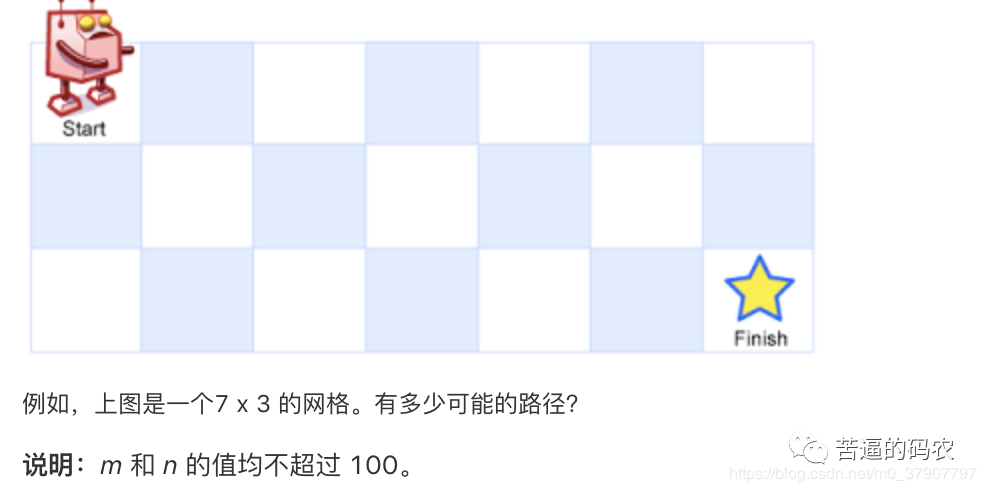# LC 狂刷 66 道 Dynamic-Programming 算法题。跟动态规划说拜拜Android架构

// 给出初始值 dp = 0;dp = 1;// 通过关系式来计算出 dp[n]for(int i = 2; i <= n; i++){dp[i] = dp[i-1] + dp[i-2];}// 把最终结果返回 return dp[n];}

（4）、再说初始化dp [0….n-1] = 1; // 相当于最上面一行，机器人只能一直往左走

dp[0…m-1]  = 1; // 相当于最左面一列，机器人只能一直往下走

public static int uniquePaths(int m, int n) {if (m <= 0 || n <= 0) {return 0;}

int[][] dp = new int[m][n]; //// 初始化 for(int i = 0; i < m; i++){dp[i] = 1;}for(int i = 0; i < n; i++){dp[i] = 1;}// 推导出 dp[m-1][n-1]for (int i = 1; i < m; i++) {for (int j = 1; j < n; j++) {dp[i][j] = dp[i-1][j] + dp[i][j-1];}}return dp[m-1][n-1];}

dp [j] = arr [j] + dp [j-1]; // 相当于最上面一行，机器人只能一直往左走

dp[i]  = arr[i]  + dp[i] ; // 相当于最左面一列，机器人只能一直往下走代码如下

public static int uniquePaths(int[][] arr) {int m = arr.length;int n = arr.length;if (m <= 0 || n <= 0) {return 0;}

int[][] dp = new int[m][n]; //// 初始化 dp = arr;// 初始化最左边的列 for(int i = 1; i < m; i++){dp[i] = dp[i-1] + arr[i];}// 初始化最上边的行 for(int i = 1; i < n; i++){dp[i] = dp[i-1] + arr[i];}// 推导出 dp[m-1][n-1]for (int i = 1; i < m; i++) {for (int j = 1; j < n; j++) {dp[i][j] = Math.min(dp[i-1][j], dp[i][j-1]) + arr[i][j];}}return dp[m-1][n-1];}

《Android学习笔记总结+最新移动架构视频+大厂安卓面试真题+项目实战源码讲义》浏览器打开：qq.cn.hn/FTe 免费领取

1 的长度为 i，字符串 word2 的长度为 j 时，将 word1 转化为 word2 所使用的最少操作次数为 dp[i] [j]。## 评论